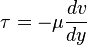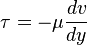# Fluid Mechanics people, little help

• wmsaqqa
Rayburn's article provides a brief overview of shear viscosity and shear strain. Shear viscosity is a measure of the resistance of a fluid to shear. Shear strain is the rate of deformation of a fluid. The shear viscosity multiplied by the shear strain will get you the shear stress.

#### wmsaqqa

What is the prove for this formula, or in another way the provement of the dynamic viscosity multiplied by the shear strain will get us the shear stress,there is no "proof" other than that many fluids conform according to this type of stress/strain rate law.

Other, non-Newtonian fluids/substances might have different force laws that are appriopriate.

I suppose it depends on what you mean by proof here. If you are familiar at all with continuum mechanics you can derive that relationship based on the assumption that you have a Newtonian fluid and a 2-D flow.

To do that, you would start out with Cauchy's equations of motion, which are
$$\nabla\cdot\mathbf{T} + \rho \vec{B} = \rho \vec{a}.$$
Here, $\mathbf{T}$ is the stress tensor, $\rho$ is the density, $\vec{B}$ is the body force vector and $\vec{a}$ is the acceleration vector. Basically this is just an expression of Newton's law. It can be rewritten in differential form:
$$\dfrac{\partial T_{ij}}{\partial x_j} + \rho B_i = \rho a_i.$$

The hydrostatic pressure in a fluid can be represented by $-p\delta_{ij}$ where $\delta_{ij}$ is the Kronecker delta, and the pressure is always going to be a part of the stress tensor and always in the diagonal terms. If the fluid is undergoing anything other than rigid body motion, there has to be another component of the stress tensor, so the stress tensor is expanded to include a term representing the stresses that will pop up in a fluid due to the rate of deformation (since a fluid is defined as having stress dependent on the rate of deformation rather than the deformation itself).
$$T_{ij} = -p\delta_{ij} + T_{ij}^{\prime}$$
where $T_{ij}^{\prime}$ represents the portion of the stress tensor that is a result of the rate of deformation of the fluid under shear. The rate of deformation is
$$D_{ij} = \dfrac{1}{2}\left( \dfrac{\partial v_i}{\partial x_j} + \dfrac{\partial v_j}{\partial x_i} \right).$$

By the definition of a Newtonian fluid, the stress due to deformation of the fluid varies linearly with the rate of deformation and the fluid is isotropic. From continuum mechanics, you can show then that
$$T_{ij}^{\prime} = \lambda\delta_{ij}D_{kk} + 2\mu D_{ijj}$$
where $\lambda$ and $\mu$ are viscosity coefficients with $\mu$ being the typical dynamic viscosity that you see all over the place. If you go look at the diagonal elements of $T_{ij}^{\prime}$, you get
$$T_{ii} = (3\lambda + 2\mu)D_{kk}.$$
Which means that $\lambda + 2\mu/3$ is a proportionality constant known as bulk viscosity relating viscous normal stresses to the rate of dilatation of the fluid. That rate, $D_{kk}$ is zero for an incompressible fluid ($\nabla\cdot\vec{v} = D_{kk} = 0$), so for an incompressible fluid, you are left simply with a stress tensor of
$$T_{ij} = -p\delta_{ij} + 2\mu D_{ij}.$$

If you go and plug this into the Cauchy equations I mentioned before, you get the Navier-Stokes equations. Otherwise, just consider a 2-D, incompressible flow with $v_1 = v_1(x_2)$ and $v_2 = v_3 = 0$. If you want to look at the shear component $T_{12}$ (the only independent nonzero component), you can simply note that
$$\tau = T_{12} = 2\mu D_{12} = 2\mu \left(\dfrac{1}{2}\dfrac{d v_1}{d x_2}\right) = \mu\dfrac{d v_1}{d x_2}.$$

This, in $(x,y)$ coordinates, is
$$\tau_{xy} = \mu\dfrac{du}{dy}.\hspace{4em}\square$$

Except for your sign convention and your typo where you swapped $u$ with $v$, that is what you have. Is this the sort of thing you were looking for?

wmsaqqa said:
What is the prove for this formula, or in another way the provement of the dynamic viscosity multiplied by the shear strain will get us the shear stress,The partial derivative in this equation is not called the shear strain. It is called the shear rate. Newton originally developed this relationship empirically from experiments he had done. Later, people generalized this to the tensorial form of the rheological relationship, in which the components of the stress tensor are linear functions of the components of the rate of deformation gradient tensor. Boneh3ad laid all the details of this relationship out in his response.

Chet